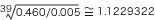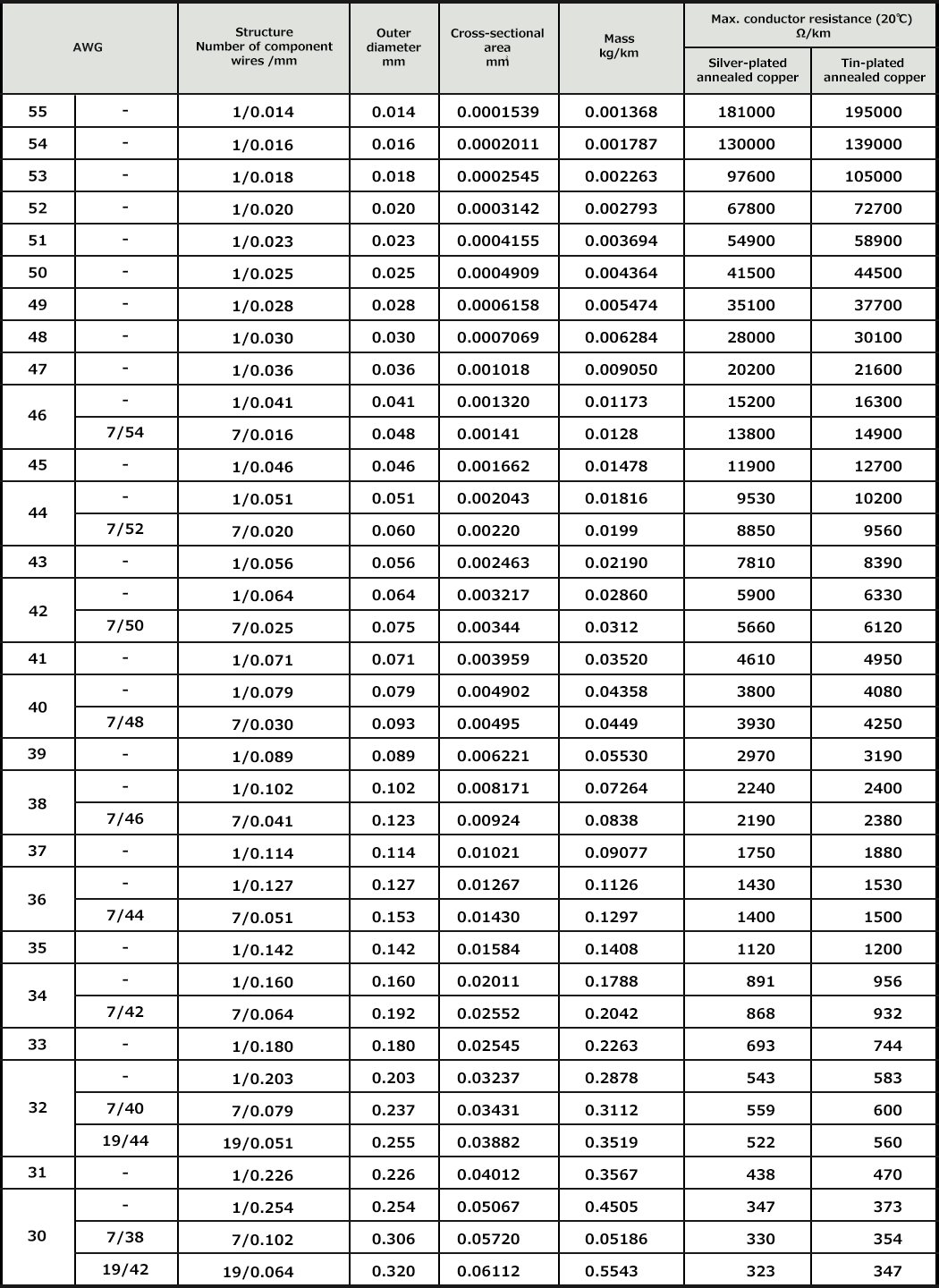## 电线电缆导体尺寸表(AWG)

### a) AWG

Abbreviation for American Wire Gauge, which is the conductor size standard commonly used in the United States. Also called B&S (Brown and Sharp) Gauge.
Given the outer diameter of 0.4600 inch for AWG No.4/0 and 0.0050 inch for AWG No.36, all gauge sizes are related to each other in geometric progression so that the interval between 0.4600 inch (4/0 AWG) and 0.0050 inch (36 AWG) is divided into 39 subintervals with a common ratio. The conductor resistance of AWG No.10 is almost 1Ω/1000-feet, and its wire diameter almost 0.1 inch.

The larger the wire No., the thinner the wire. The model No., conductor resistance, and weight are related as follows:
If wire No. increases by three, conductor resistance doubles and weighs 1/2, approximately.
If wire No. increases by six, conductor resistance increases fourfold, weighs 3/4 less, and diameter decreases by 1/2 (approximately).
If wire No. increases by ten, conductor resistance increases tenfold, weighs 9/10 less, and the cross-sectional area decreases by 9/10 (approximately).

AWG is used in MIL-compliant and UL-compliant products. The specs for each size of wire prescribe the dimensional tolerance and conductor resistance.
The American Society for Testing and Materials (ASTM) outlines individual wire structure and test methods.
In AWG, the cross-sectional area is often expressed in circular mils (CMs). The area of a circle with a diameter of 1 mil (= 0.001 inch = 0.0254m) is 1 CM. The following table is useful for unit conversion.

Square millimeter Circular mil Square inch
Square millimeter 1 1973.5 0.001550
Circular mil 0.00050671 1 0.7854x10-6
Square inch 645.16 1.2732x106 1
1mm2 = 1973.5 CM = 0.001550 square inches
Conductor Size Tables (Table 1-1-1)
1. Mass is calculated from the specific gravity of annealed copper (8.89).
2. The conductor resistance is the maximum specified value of a single conductor measured at 20℃. For stranded cable, the conductor resistance is multiplied by the stranding factor.
3. When calculating the electric resistance of a conductor of different conductivity, multiply the resistance of silver-plated annealed copper by (100/conductivity).
4. Not all sizes of conductor (plated or alloys) in the tables are available. Contact us for available sizes or types not listed in the table or contained in Junkosha’s catalog.
5. In terms of conductor resistance, give priority to any other values defined in individual cable specs (e.g., MIL, UL).
Table 1-1-1 AWG Conductor Size